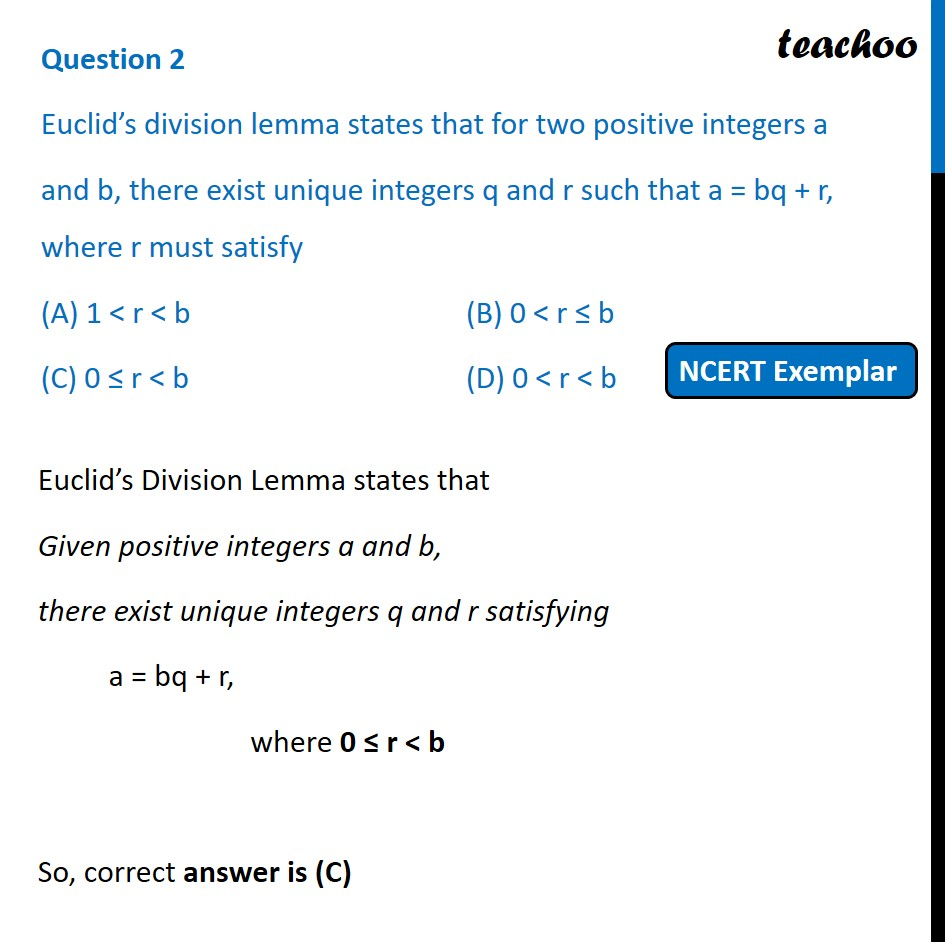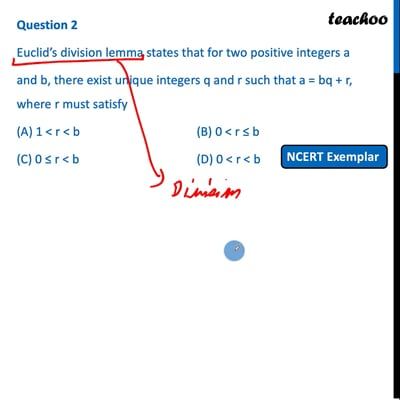MCQs from NCERT Exemplar

Chapter 1 Class 10 Real Numbers
Serial order wise

## (C) 0 ≤ r < b   (D) 0 < r < bThis video is only available for Teachoo black users

Introducing your new favourite teacher - Teachoo Black, at only ₹83 per month

### Transcript

Question 2 Euclid’s division lemma states that for two positive integers a and b, there exist unique integers q and r such that a = bq + r, where r must satisfy (A) 1 < r < b (B) 0 < r ≤ b (C) 0 ≤ r < b (D) 0 < r < b Euclid’s Division Lemma states that Given positive integers a and b, there exist unique integers q and r satisfying a = bq + r, where 0 ≤ r < b So, correct answer is (C)